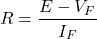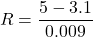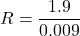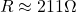# Ballast Resistor: What is it? (Types of Ballast Resistors And How To Test Them)

Contents

## What is a Ballast Resistor?

A ballast resistor is defined as a resistor inserted into a circuit to minimize current. Ballast resistors also help to avoid over-current faults in a circuit. An “electric ballast” is a more general term used to refer to an electrical device used to maintain a circuit’s stability by limiting the value of current and voltage. Electric ballasts can be resistors, capacitors, inductors, or a combination of these.

Ballast resistors are able to change resistance with the current. If the current flowing through the resistor increases above the threshold value, the resistance increases. The resistance can then correspondingly decrease as the current decreases.

In this way, the ballast resistor tries to maintain a constant current flowing through a circuit.

The ballast resistor is different from the load resistor. As it acts like a variable load connected with the system. But in the case of load resistor, resistance remains constant with different values of current and voltage.

Ballast resistors are no longer widely used. They have been replaced by electronic circuits that fulfill the same function.

## What does a Ballast Resistor do?

The term “ballast” relates to stability. Therefore, when we use the term ballast resistor, it refers to the fact that the ballast resistor is helping maintain the stability of the electrical circuit.

A ballast resistor is used in a device to compensate for changes and secure the other components of a network.

When the current flowing through the resistor increase, the temperature also increases. And due to the temperature rise, the resistance also increases.

Therefore, an increase in resistance limits the current flowing through the network.

Ballast resistors are widely used in automotive applications to start the engine. When the starter motor starts the engine, the ballast register limits voltage drain from the battery.

It is also used in the lighting applications such as a fluorescent lamp, LED, and neon lights.

## Applications of a Ballast Resistor

A ballast resistor helps to regulate the current and voltage in an electrical system. It helps equipment from experiencing overcurrent and overvoltage events.

Ballast resistors are mostly used in automotive and lighting applications.

### Ballast Resistor for Automotive Applications

In a car engine, a ballast resistor is used in the ignition system. And it is known as an ignition ballast resistor.

Generally, an ignition ballast resistor is placed between the primary source of the ignition coil and coil stud. It reduces the failure risk of the ignition coil.

When the starter motor cranks the engine, the ignition ballast resistor helps to reduce the coil voltage and coil current.

Therefore, low current causes a low-temperature rise. And it results in a long life of the ignition coil.

But the ignition system needs a higher voltage which is equal to the voltage of the power source. So, the jumper wire is connected with an ignition ballast resistor. And while starting the engine, the jumper wire provides the required voltage to the ignition coil.

### Ballast Resistor in a LED Circuit

The LED (Light Emitting Diode) is a very sensitive device. It may damage if the supply voltage is more than the rated voltage.

To avoid such conditions, a ballast resistor is used to connect in series with the LED. It will reduce the voltage across the LED to its rated value.

We need to connect the appropriate resistance value of ballast resistance. For that consider the below example.

Consider a single LED is connected in series with the supply source. Here, the value of the supply source is higher than the rated voltage. Therefore, you cannot connect directly without a resistor.Where;

VF = Forward voltage of LED

IF = Forward current of LED

R = Resistance of Ballast resistor

E = Supply voltage

For example, the value of DC source is 5V. The forward rated voltage of LED is 3.1V and forward current is 9mA. From the above equation;Hence, for this example, we need to connect a 211Ω or more than this rating of the resistor.

## Types of Ballast Resistors

The ballast resistors are classified into two types;

• Fixed Ballast Resistor
• Self-variable Ballast Resistor

### Fixed Ballast Resistor

As the name suggests, the resistance of this type of resistor is fixed. This type of ballast resistor is generally only used in simple circuits.

Fixed ballast resistors used in various applications where a high resistance value is preferred.

For example, it is used in a circuit with a neon lamp or LED. It is also used in the variable speed fan.

In a variable-speed fan, a fixed ballast resistor with two center taps is used. The fan selector switch sets the value of resistance with the help of the center tap. And according to the value of resistance, the speed of a fan varies.

### Self-variable Ballast Resistor

This type of resistor has a property to change its resistance with changes in current. When the current flowing through the resistor increase, it increases the temperature of the ballast resistor. As a result, the resistance also increases.

This type of ballast resistor is used in an incandescent lamp. When the current passing through the lamp increase, the resistance of the ballast resistor increases. And it also increases the voltage drop across the resistor.

When the current decreases, the temperature goes down and resistance decreases. This type of resistance is also used to protect circuit equipment against overcurrent.

## How to Test a Ballast Resistor

The ballast resistor is used in automotive applications. It is used to reduce the voltage level of the ignition coil. To test the ballast resistor, we need an ohmmeter and multimeter.

If the ballast resistor is not connected with the ignition coil, the full supply voltage is applied to the ignition coil.

Generally, the battery is used to supply the ignition system. And in most cases, the battery voltage will be 12V or 24V.

To increase the life of the ignition coil, we need to reduce voltage. and to fulfill this purpose, we connect a ballast resistor with the ignition coil.

So, to test the ballast resistor, we can measure the voltage across the ignition coil.

If the ballast resistor is good, it reduced the voltage level to 7-8V. If the ballast is damaged, the voltage level of the ignition coil will be high.

We can check the resistance of the ballast resistor with the help of an ohmmeter. If it is near the rated resistance, this is a good sign that the ballast resistor is in a healthy condition.

Want To Learn Faster? 🎓
Get electrical articles delivered to your inbox every week.
No credit card required—it’s 100% free.